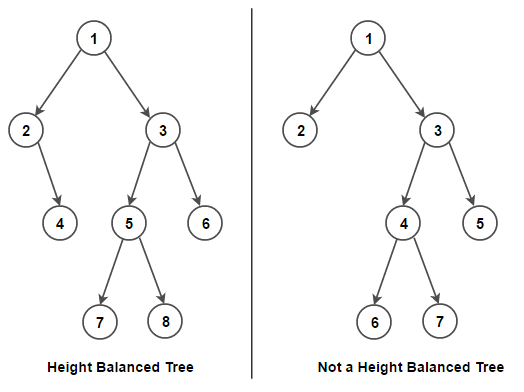# Check if given Binary Tree is Height Balanced or not

Given a binary tree, write an efficient algorithm to check if tree is height balanced or not. In a height balanced tree, the absolute difference between height of left subtree and right subtree for every node is 0 or 1.

For example,Simple solution would be to calculate the height of left subtree and right subtree for each node in the tree. If for any node, the absolute difference between the height of its left and right subtree is more than 1, the tree is unbalanced. The time complexity of this solution is O(N2) as there are N nodes in the tree and for every node we are calculating height of its left subtree and right subtree that takes O(N) time.

We can solve this problem in linear time by doing post-order traversal of the tree. Instead of calculating height of left subtree and right subtree for every node in the tree, we can get the height in constant time. The idea is to start from the bottom of the tree and return height of subtree rooted at given node to its parent node. The height of subtree rooted at any node is equal to 1 plus maximum height of the left subtree or right subtree.

C++ implementation –

The time complexity of above solution is O(n) and auxiliary space used by the program is O(1).(1 votes, average: 5.00 out of 5)Loading...

Please use our online compiler to post code in comments. To contribute, get in touch with us.
Like us? Please spread the word and help us grow. Happy coding 🙂